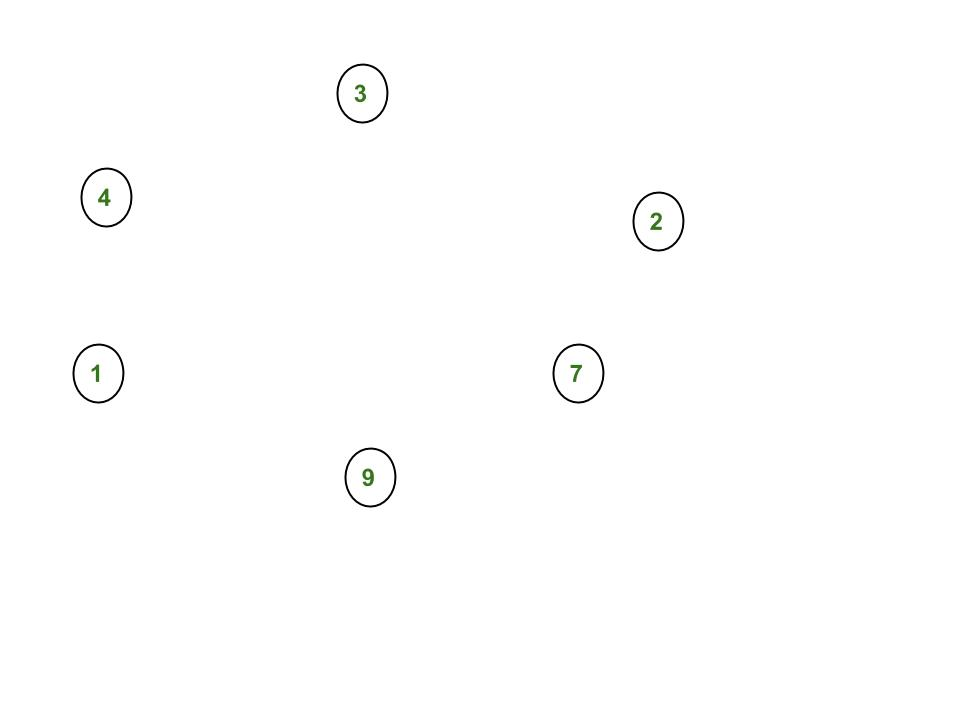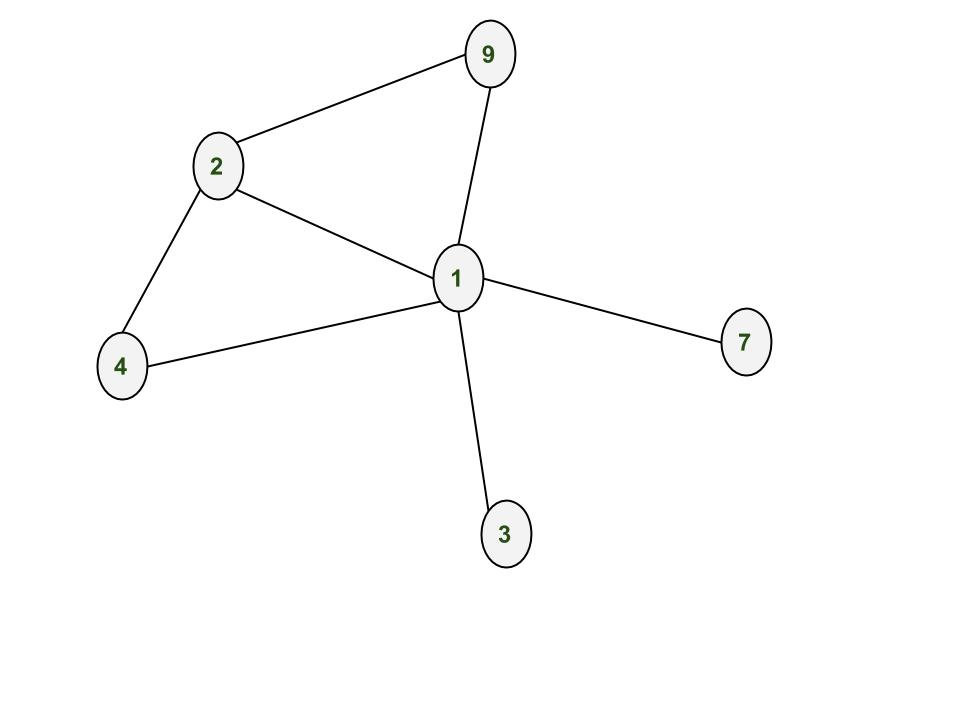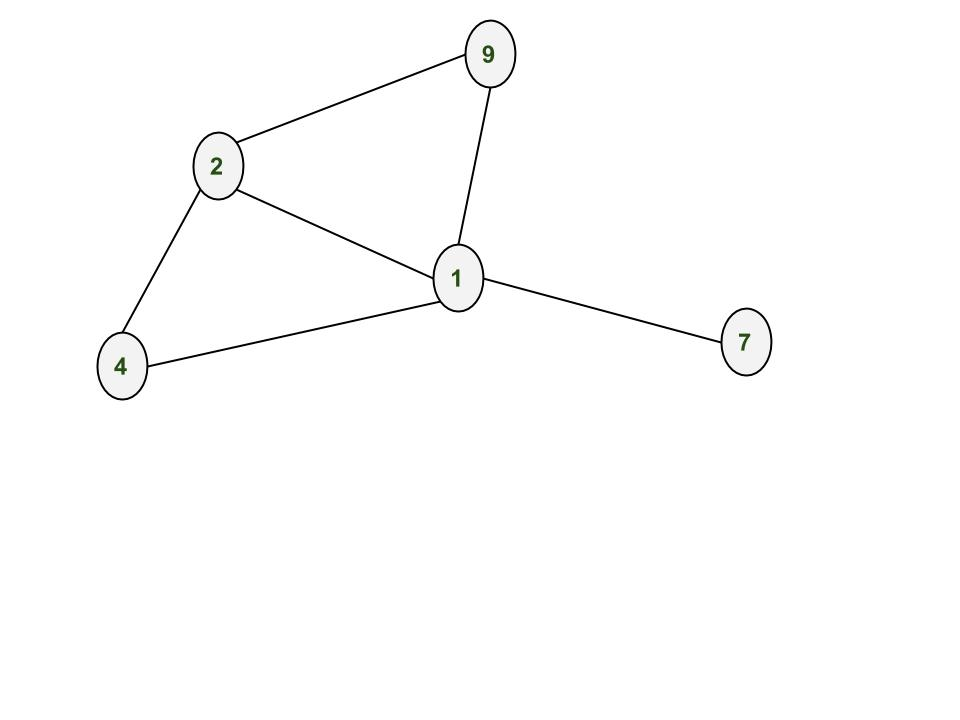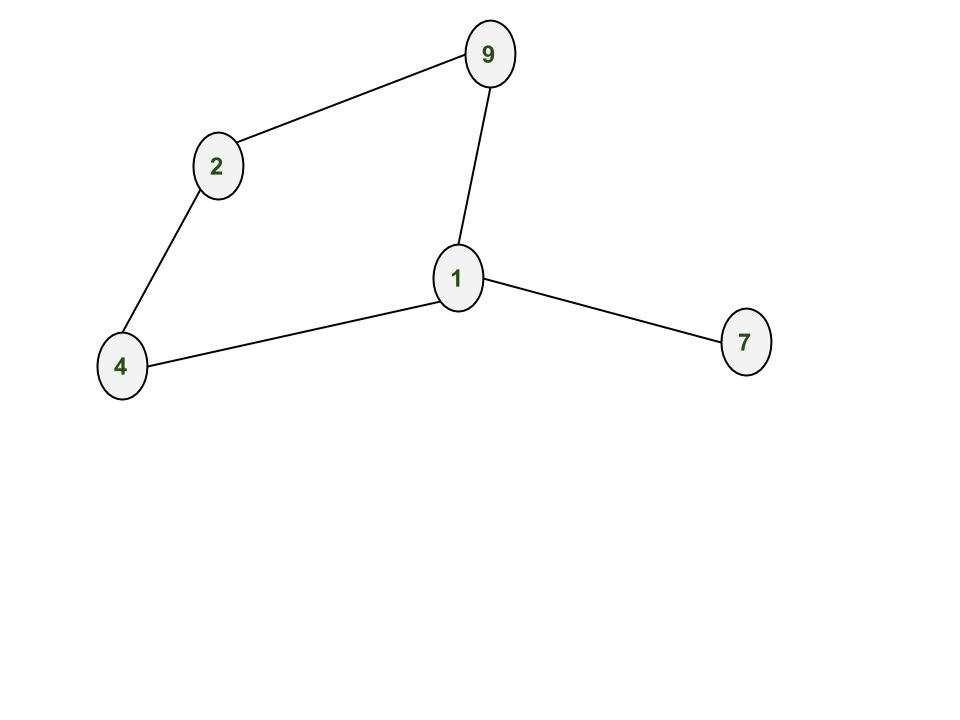# NetworkX : Python software package for study of complex networks

NetworkX is a Python language software package for the creation, manipulation, and study of the structure, dynamics, and function of complex networks. It is used to study large complex networks represented in form of graphs with nodes and edges. Using networkx we can load and store complex networks. We can generate many types of random and classic networks, analyze network structure, build network models, design new network algorithms and draw networks.

Installation of the package:

`pip install networkx`

Creating Nodes

Add one node at a time:

` G.add_node(1)`

` G.add_nodes_from([2,3])`

Let us create nodes in the graph G. After adding nodes 1, 2, 3, 4, 7, 9Creating Edges:

Adding one edge at a time:

```G.add_edge(1,2)

`G.add_edges_from([(1,2),(1,3)])`

After adding edges (1,2), (3,1), (2,4), (4,1), (9,1), (1,7), (2,9)Removing Nodes and Edges:

One can demolish the graph using any of these functions:

```Graph.remove_node(), Graph.remove_nodes_from(),
Graph.remove_edge() and Graph.remove_edges_from()```

After removing node 3After removing edge (1,2)## Python3

 `# Python program to create an undirected ` `# graph and add nodes and edges to a graph`   `# To import package` `import` `networkx` ` `  `# To create an empty undirected graph` `G ``=` `networkx.Graph()` ` `  `# To add a node` `G.add_node(``1``)` `G.add_node(``2``)` `G.add_node(``3``)` `G.add_node(``4``)` `G.add_node(``7``)` `G.add_node(``9``)` ` `  `# To add an edge` `# Note graph is undirected` `# Hence order of nodes in edge doesn't matter` `G.add_edge(``1``,``2``)` `G.add_edge(``3``,``1``)` `G.add_edge(``2``,``4``)` `G.add_edge(``4``,``1``)` `G.add_edge(``9``,``1``)` `G.add_edge(``1``,``7``)` `G.add_edge(``2``,``9``)` ` `  `# To get all the nodes of a graph` `node_list ``=` `G.nodes()` `print``(``"#1"``)` `print``(node_list)` ` `  `# To get all the edges of a graph` `edge_list ``=` `G.edges()` `print``(``"#2"``)` `print``(edge_list)` ` `  `# To remove a node of a graph` `G.remove_node(``3``)` `node_list ``=` `G.nodes()` `print``(``"#3"``)` `print``(node_list)` ` `  `# To remove an edge of a graph` `G.remove_edge(``1``,``2``)` `edge_list ``=` `G.edges()` `print``(``"#4"``)` `print``(edge_list)` ` `  `# To find number of nodes` `n ``=` `G.number_of_nodes()` `print``(``"#5"``)` `print``(n)` ` `  `# To find number of edges` `m ``=` `G.number_of_edges()` `print``(``"#6"``)` `print``(m)` ` `  `# To find degree of a node` `# d will store degree of node 2` `d ``=` `G.degree(``2``)` `print``(``"#7"``)` `print``(d)`   `# To find all the neighbor of a node` `neighbor_list ``=` `G.neighbors(``2``)` `print``(``"#8"``)` `print``(neighbor_list)`   `#To delete all the nodes and edges` `G.clear()`

Output:

```#1
[1, 2, 3, 4, 7, 9]
#2
[(1, 9), (1, 2), (1, 3), (1, 4), (1, 7), (2, 4), (2, 9)]
#3
[1, 2, 4, 7, 9]
#4
[(1, 9), (1, 4), (1, 7), (2, 4), (2, 9)]
#5
5
#6
5
#7
2
#8
[4, 9]```

In the next post, we’ll be discussing how to create weighted graphs, directed graphs, multi graphs. How to draw graphs. In later posts we’ll see how to use inbuilt functions like Depth first search aka dfs, breadth first search aka BFS, dijkstra’s shortest path algorithm.

If you like GeeksforGeeks and would like to contribute, you can also write an article using write.geeksforgeeks.org or mail your article to review-team@geeksforgeeks.org. See your article appearing on the GeeksforGeeks main page and help other Geeks.

Feeling lost in the world of random DSA topics, wasting time without progress? It's time for a change! Join our DSA course, where we'll guide you on an exciting journey to master DSA efficiently and on schedule.
Ready to dive in? Explore our Free Demo Content and join our DSA course, trusted by over 100,000 geeks!